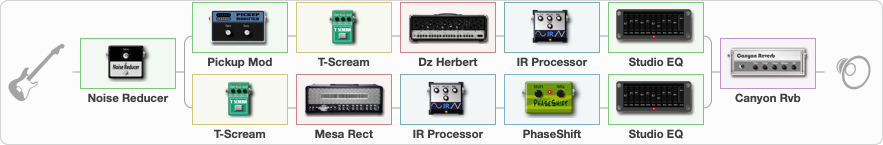# KH MASTER OF PUPPETS

Discussion in 'ToneLib-GFX presets' started by grigoriy0192873, Mar 20, 2023.

1. ### grigoriy0192873Active Member

KH MASTER OF PUPPETS

Preset name: KH MASTER OF PUPPETS

Effects chain:Effect: "Noise Reducer" (Dynamics / Filter), active - "yes"
{
"Sens" = 65
"Mode" = Hard
}

Effect: "Splitter" (Dynamics / Filter)
{
"A-Bypass" = Off
"A-Pan" = 65
"A-Level" = 66
"B-Bypass" = Off
"B-Pan" = -65
"B-Level" = 66
"Width" = 0

'A' branch:
{

Effect: "Pickup Mod" (Dynamics / Filter), active - "yes"
{
"Mode" = Phase Out
"Tone" = 50
}

Effect: "T-Scream" (Overdrive / Distortion), active - "yes"
{
"Drive" = 24
"Tone" = 71
"Level" = 82
}

Effect: "Dz Herbert" (Amp simulators), active - "yes"
{
"Gain" = 50
"Bass" = 50
"Middle" = 0
"Treble" = 73
"Presence" = 50
"Master" = 50
"Level (dB)" = 4
}

Effect: "IR Processor" (Cabinets), active - "yes"
{
"IR" = Puppet_IR
"Low Cut (Hz)" = 0
"Hi Cut (kHz)" = 20.0
"Mix" = 100
"Level (dB)" = 0
}

Effect: "Studio EQ" (Dynamics / Filter), active - "yes"
{
"31 Hz" = 0
"62 Hz" = 0
"125 Hz" = 0
"250 Hz" = -5
"500 Hz" = -12
"1 kHz" = -5
"2 kHz" = 5
"4 kHz" = 3
"8 kHz" = 3
"16 kHz" = 0
"Level (dB)" = 0
}
}
'B' branch:
{

Effect: "T-Scream" (Overdrive / Distortion), active - "yes"
{
"Drive" = 32
"Tone" = 71
"Level" = 82
}

Effect: "Mesa Rect" (Amp simulators), active - "yes"
{
"Gain" = 50
"Bass" = 50
"Middle" = 0
"Treble" = 73
"Presence" = 50
"Master" = 50
"Level (dB)" = 3
}

Effect: "IR Processor" (Cabinets), active - "yes"
{
"IR" = Puppet_IR
"Low Cut (Hz)" = 0
"Hi Cut (kHz)" = 20.0
"Mix" = 100
"Level (dB)" = 0
}

Effect: "PhaseShift" (Modulation / Sfx), active - "yes"
{
"Shift" = 55
"Mix" = 100
}

Effect: "Studio EQ" (Dynamics / Filter), active - "yes"
{
"31 Hz" = 0
"62 Hz" = 0
"125 Hz" = 0
"250 Hz" = -5
"500 Hz" = -12
"1 kHz" = -5
"2 kHz" = 5
"4 kHz" = 3
"8 kHz" = 3
"16 kHz" = 0
"Level (dB)" = 0
}
}
}

Effect: "Canyon Rvb" (Reverberation), active - "yes"
{
"Time" = 6.1
"PreDelay" = 42
"LoDamp" = 0
"HiDamp" = 32
"Mix" = 39
}

Note: You will need to download and install the ToneLib-GFX software to use the preset.

File size:
4.8 KB
Views:
1,658
File size:
4.8 KB
Views:
6